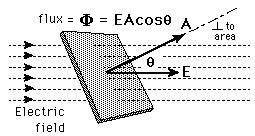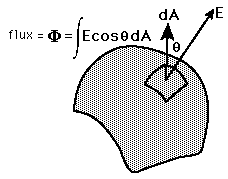Tests

Homework Exercises Solutions

Math 2AMultivariate CalculusFall 2007# Vector Calculus Links

Xah Lee has a collection of interesting vector calculus related info. The Virtual Math Museum is interesting. He's got a page of great math softwares that includes many interesting links.  Try the SpaceCurveExplorer and The ParametricSurfaceViewer applets on the Virtual Labs page.

On his TechBlog, Yanto Suryano has posted an Surface Plotter applet for plotting surfaces that's simple and to the point.  He includes the source code, if you're interested in that.

Jeff Knisely's applet is nice, except it doesn't always work (?):

http://butlercc.edu/mathematics/Utilities/tools_multivariable_knisley/

Nestled in the charming village of South Orange, New Jersey, Seton Hall University is only 14 miles from New York City, one of the world's most exciting cities and has a fine Math and Computer Science program.Their plotter is very nice, except that while you drag the surface plot, it disappears.

http://sciris.shu.edu/thinklets/Math/Calculus/SurfacePlotter/Surface.html

MIT's math department has a collection of calculus pages here you can get into from here:
http://math.mit.edu/cgi-bin/classes.cgi .  Click on 18.02 for a collection of links to various vector calculus related documents.  You can also access the pull down box at the top to choose another term and a differential equations link such as http://www-math.mit.edu/18.013A/HTML/index.html

David Little at Penn State has a nice space curve applet you can also download locally, they say.  It shows little plane panels of the three x, y and z functions in windows at the top.
http://www.math.psu.edu/dlittle/java/calculus/spacecurves.html

Matthias Kowski at Arizona State University set up this interesting applet: a Vector Field Analyzer which can really help build your intuitive sense of vector fields and their equations.  Here are some links:  http://math.la.asu.edu/~kawski/vfa2/index.html

Using Maple.

http://omega.albany.edu:8008/calc3/toc.html

http://math.etsu.edu/MultiCalc/intro/intro2.htm
====

Compare the derivation of how to find the osculating plane in example 7 of 10.3 in the Concepts and Context book found here
http://www.stewartcalculus.com/data/ESSENTIAL%20CALCULUS%20Early%20Transcendentals/upfiles/examples/ess_ae_1008.pdf
with this explanation of how to solve the same problem:

========================

Limits